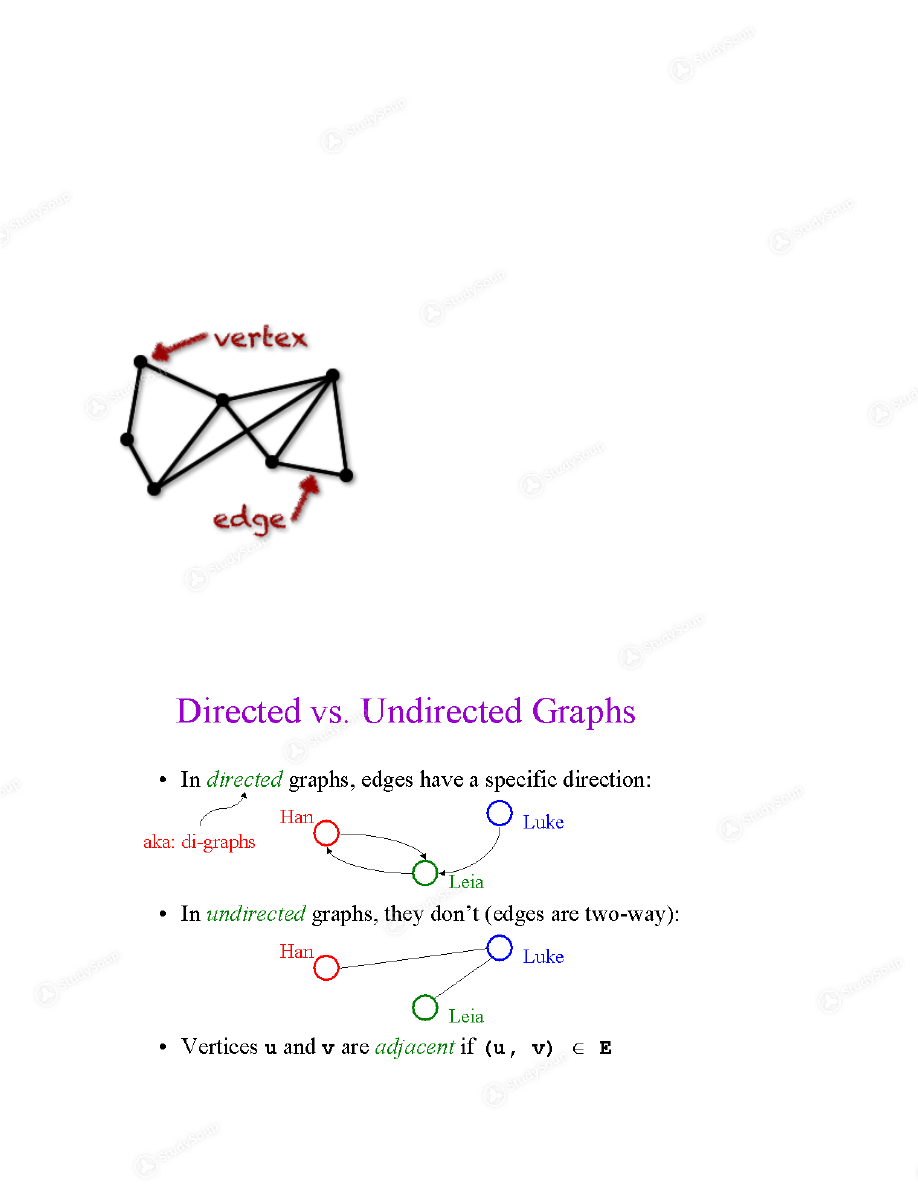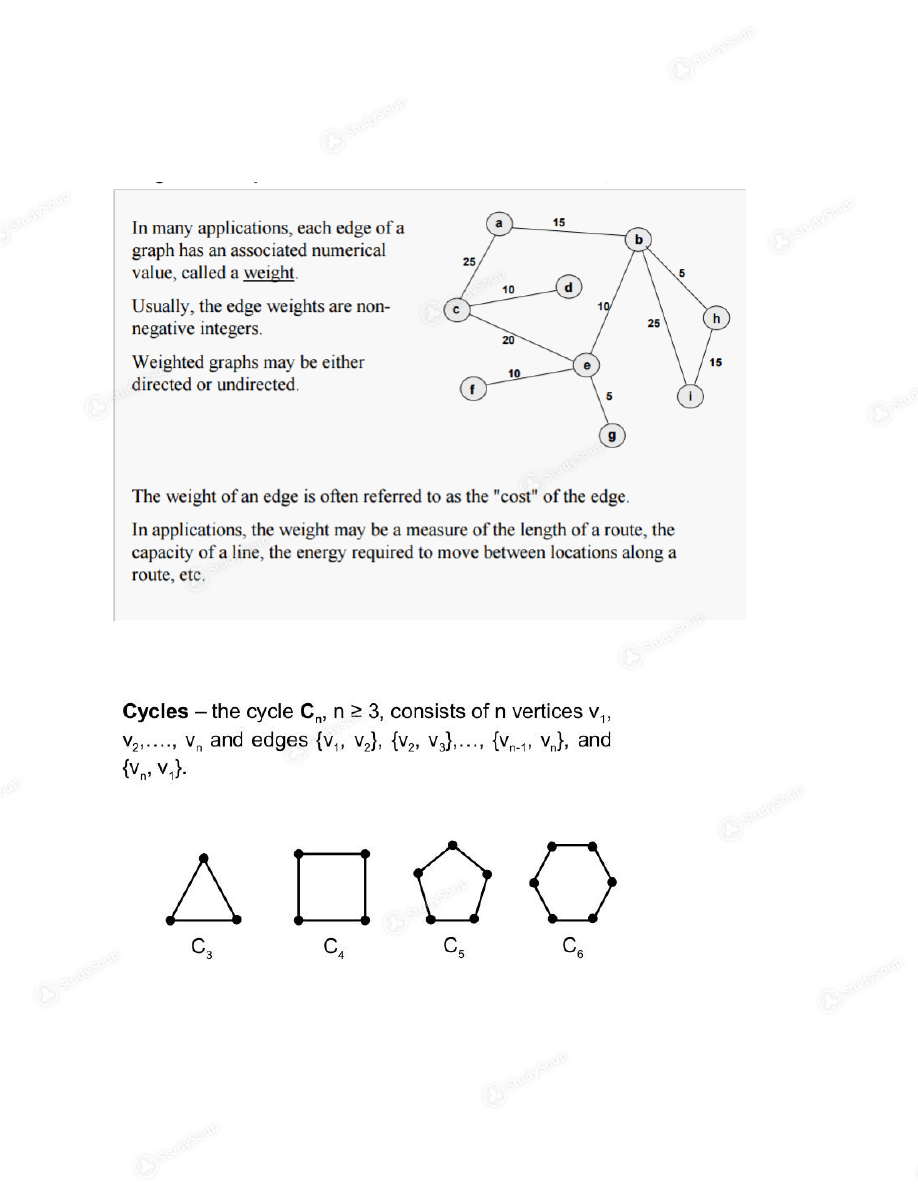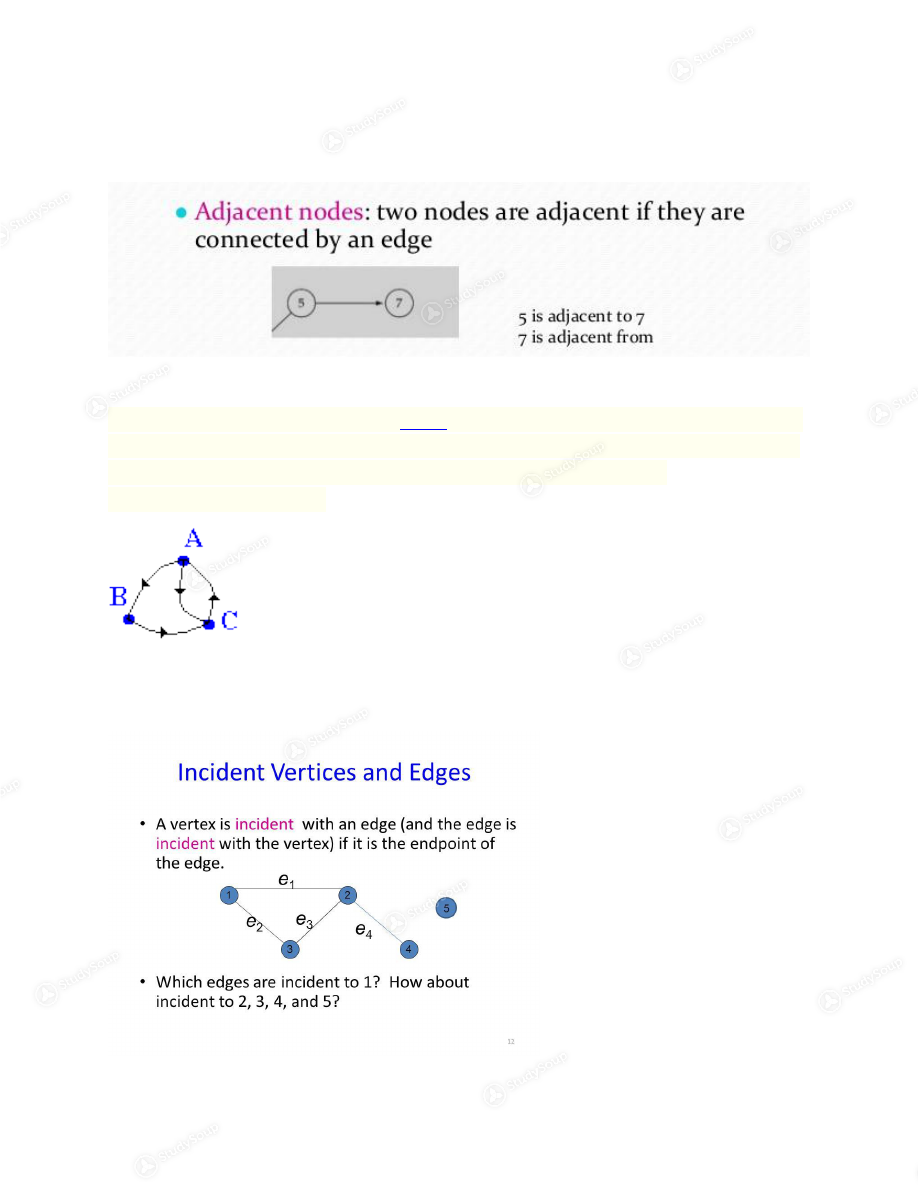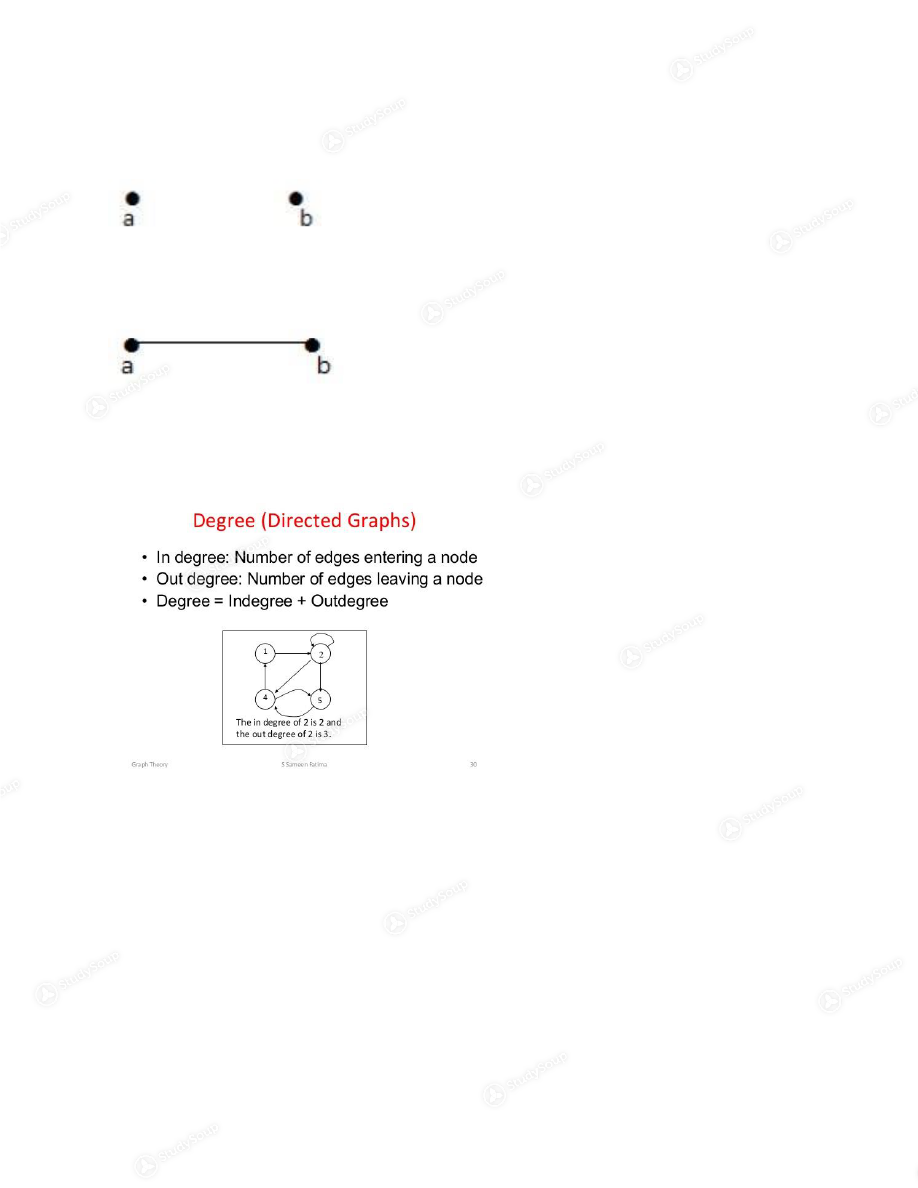×

# PSU - CS 250 - CS 250, Final Study Guide - Study Guide

### Created by: Parker Moore Elite Notetaker

> > > > PSU - CS 250 - CS 250, Final Study Guide - Study Guide

PSU - CS 250 - CS 250, Final Study Guide - Study Guide

0 5 3 18 Reviews
This preview shows pages 1 - 4 of a 19 page document. to view the rest of the contentCS250, Week 10 Notes  PSU: Fall term    Graph Theory:   A graph is a pair (V, E), where V is a set of elements called vertices, nodes, or points
and E is a set of pairs of vertices called edges or arcs.
Variations:   Edges can be directed or undirected.Edges can be weighted.     Edges can cycle:Notations:  Adjacent nodes    Initial and terminal node of an edge  A digraph (or a directed graph) is a  graph  in which the edges are directed. (Formally:  a digraph is a (usually finite) set of vertices V and set of ordered pairs (a,b) (where a,
b
are in V) called edges. The vertex a is the initial vertex of the edge
and b the terminal vertex
Edge incident to and from a nodeDegrees of vertex, isolated (0), pendant (1)  A vertex with degree zero is called an isolated vertex.    By using degree of a vertex, we have a two special types of vertices. A vertex
with degree one is called a pendent vertex.
In-degree deg-(v) and out degree deg+(v) of node v    Adjacency matrix:  In graph theory and computer science, an adjacency matrix is a square matrix used to
represent a finite graph. The elements of the matrix indicate whether pairs of vertices are
adjacent or not in the graph. In the special case of a finite simple graph, the adjacency
matrix
is a (0,1)-matrix with zeros on its diagonal.

This is the end of the preview. Please to view the rest of the contentJoin more than 18,000+ college students at Portland State University who use StudySoup to get ahead